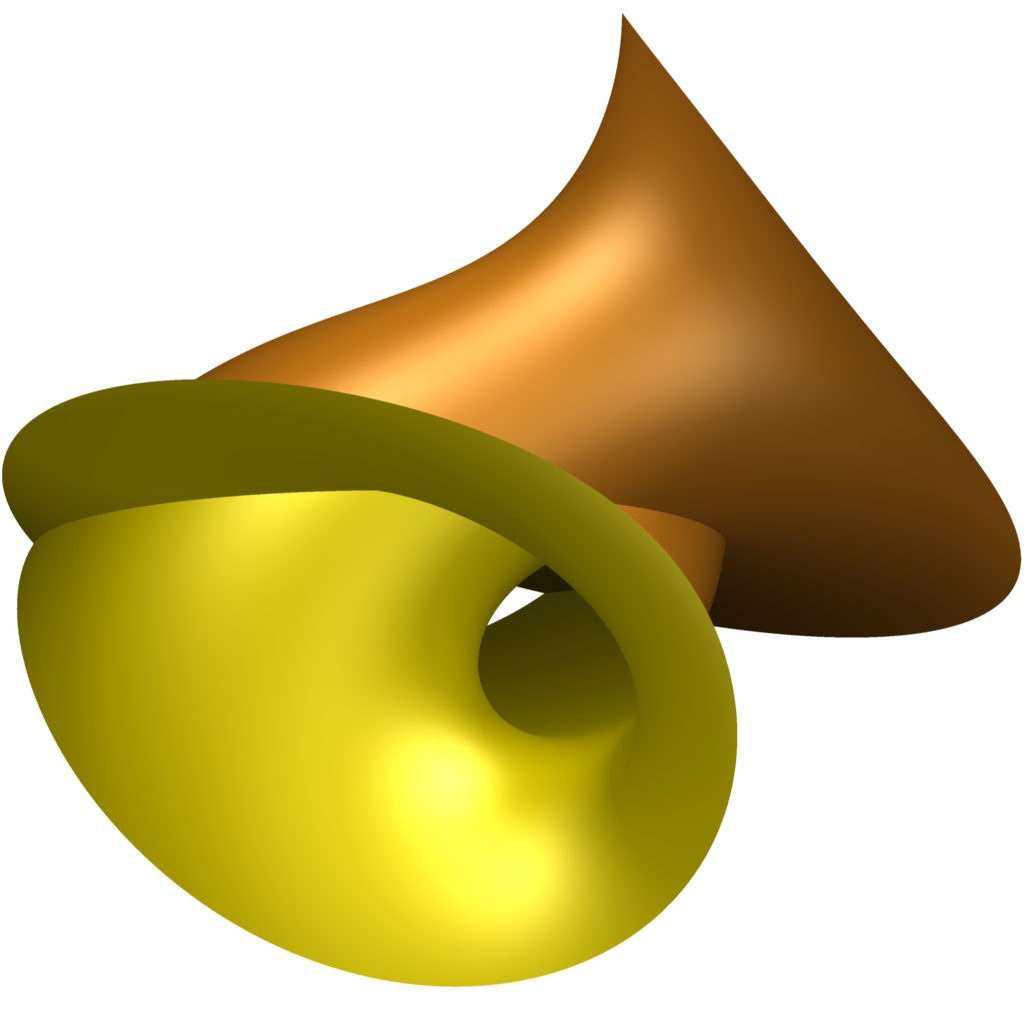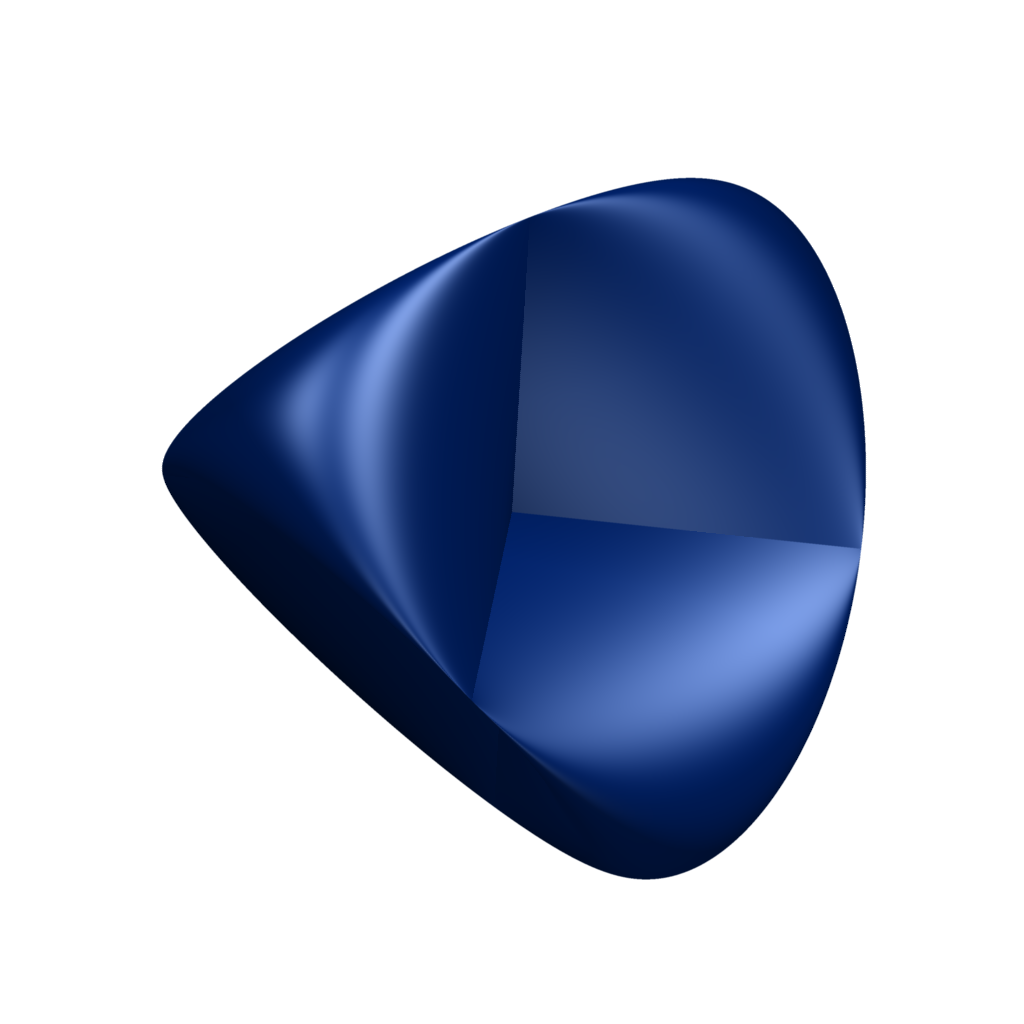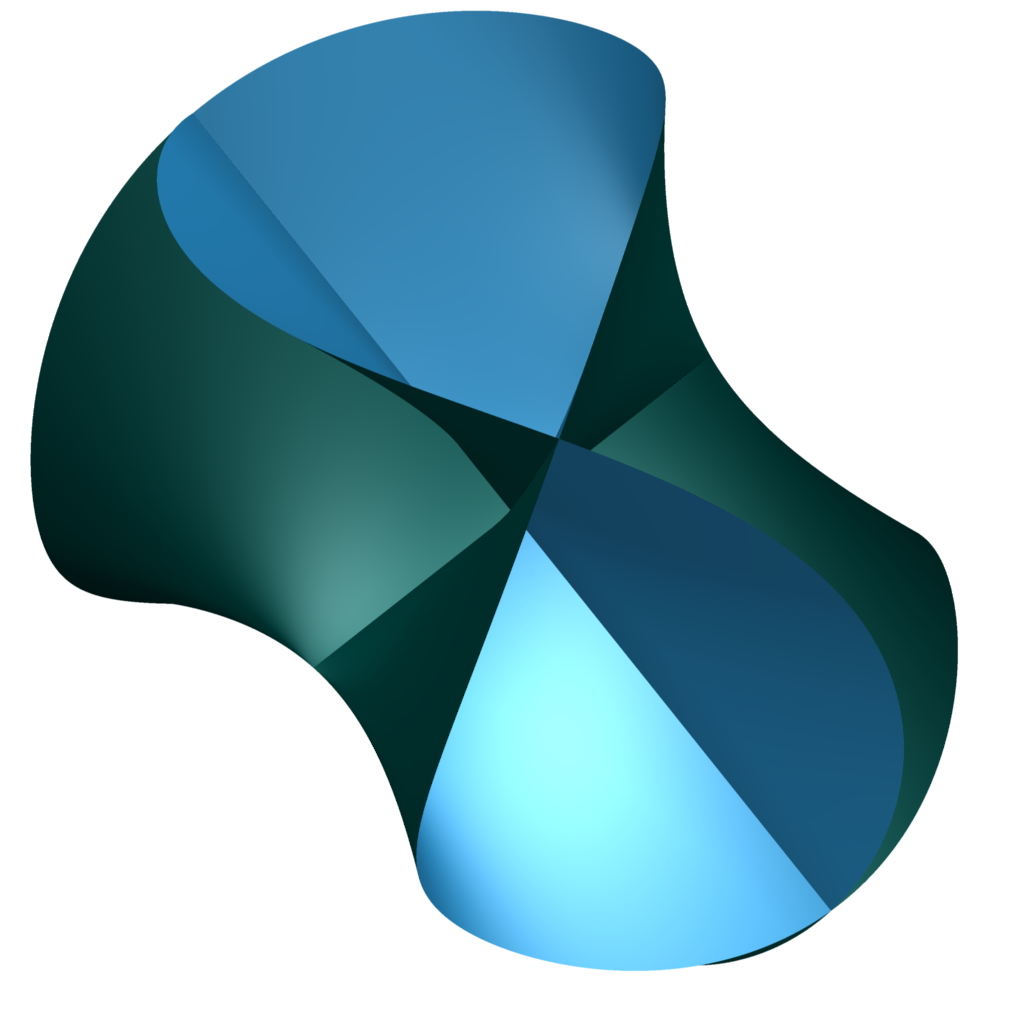# Algebraic surface toys!

Published: Fri 25 January 2008

At the start of the German Year of Mathematics, the Oberwolfach research institute has released an exhibition and the software they used to produce it. The software, surfer, is a really nice GUI that sits on top of surf and lets you rotate and zoom your algebraic surfaces as well as pick colours very comfortably.

They have a whole bunch of Really Pretty Images at the exhibition website, and I warmly recommend a visit. If you can get hold of the exhibition, they also have produced real models - with a 3d-printer - of some of the snazzier surfaces, so that one could have a REALLY close encounter with them.

But also, I'd really like to show you some of my own minor experiments with the program.This is the interior of a Klein Bottle, using the "standard" realization as an algebraic surface given by Mathworld. In other words, I'm using
(x^2+y^2+z^2+2*y-1)*((x^2+y^2+z^2-2*y-1)^2-8*z^2)+16*x*z*(x^2+y^2+z^2-2*y-1)=0
for the defining equation. It kinda looks a bit like a Sousaphone in my opinion.Roman's surface, an immersion of the real projective plane into 3-dimensional euclidean space. It is given by the equation
(x^2+y^2+z^2-9)^2-((z-3)^2-2*x^2)*((z+3)^2-2*y^2)=0
and is one of the Steiner projections of the Veronese surface, embedding the real projective plane into projective 5-dimensional space by the homogenous parametrization (x^2,y^2,z^2,xy,xz,yz).With the defining equation
x^2*y^2-x^2*z^2+y^2*z^2-x*y*z=0
this Steiner surface can be transformed into the Roman surface above if (and only if) you're allowed to take shortcuts over the points at infinity. As it is, it has two pinches (both visible) and three lines of self-intersections (also all visible, kinda sorta). It's also unbounded - one of the reasons that you cannot get to the bounded Roman surface easily.

With this as inspiration - go forth and draw surfaces. And when you do, please show them to me too.